Deutsche VersionGeometric Mean and Arithmetic Mean

Calculation of the geometric mean of two numbers ●
e.g. calculating the center frequency f0 of a bandwidth BW = f2f1

Comparison between the arithmetic mean (average) and the geometric mean

Formula: Difference between arithmetic average and geometric average.

You cannot calculate the geometric mean from the arithmetic mean.

Enter the numbers f1 and f2 in the boxes, click thecalculation button, and compare the two answers.

 f1 Hz f2 Hz ↓ Geometric mean f0 Hz Arithmetisches Mittel f0 Hz
 The geometric mean between two numbers (formula):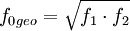The arithmetic mean between two numbers is: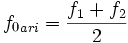Example: The cut-off frequencies of a phone line are f1 = 300 Hz and f2 = 3300 Hz. What is the center frequency?   The center frequency is f0 = 995 Hz as geometric mean and not f0 = 1800 Hz as arithmetic mean. What a big difference!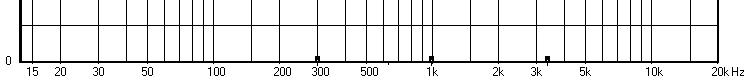f1                           f0                          f2
 Telephone transmission range as logarithmic scale. The center frequency is 995 Hz. The linear distance from 300 Hz to 995 Hz is equal to the linear distance from 995 Hz to 3300 Hz. The frequency range 300 Hz to 3.3 kHz is the bandwidth of the transmission of 3 kHz. Sometimes the phone transmission goes even up to 3.4 kHz.The HiFi range goes from f1 = 20 Hz to f2 = 20000 Hz. The correct center frequency is f0 = 632.5 Hz (!) as geometric mean and not the value 10.01 kHz of the arithmetic mean calculation. Look here: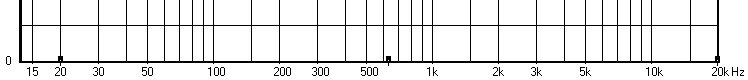f1                                                                               f0                                                                                f2
 Audible frequency range with a logarithmic scale. The linear distance from 20 Hz to 632 Hz is equal to the linear distance from 632 Hz to 20 kHz. Look at the marked points in the figure.

 By defining the center frequency, the ratios of the cut-off frequencies to the center frequency are the same: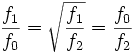The geometric mean of two numbers is the square root of their product. The geometric mean of three numbers is the cubic root of their product.  The arithmetic mean is the sum of the numbers, divided by the quantity of the numbers. Other names for arithmetic mean: average, mean, arithmetic average.   In general, you can only take the geometric mean of positive numbers.

 The geometric mean, by definition, is the nth root of the product of the n units in a data set. For example, the geometric mean of 5, 7, 2, 1 is (5 × 7 × 2 × 1)1/4 = 2.893. Alternatively, if you log transform each of the individual units the geometric will be the exponential of the arithmetic mean of these log-transformed values. So, reusing the example above, exp [ ( ln(5) + ln(7) + ln(2) + ln(1) ) / 4 ] = 2.893.

Geometric Mean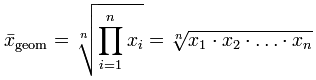Arithmetic Mean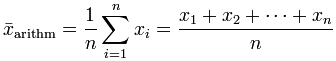Calculating the -3 dB cut-off frequencies f1 and f2 when center frequency and Q factor is given.

Geometric mean of more numbers

Calculate Geometric Mean
Enter all the numbers separated by comma,
e.g. 300,3000

 Total numbers: Geometric mean:

 Geometric Mean Tutorial   Geometric Mean Definition: Geometric Mean is a kind of average of a set of numbers that is different from the arithmetic average. The geometric mean is well defined only for sets of positive real numbers. This is calculated by multiplying all the numbers (call the number of numbers n), and taking the nth root of the total. A common example of where the geometric mean is the correct choice - is when averaging growth rates.   Formula: Geometric Mean = ((x1)(x2)(x3) ... (xn))1/n   where x = Individual score and n = Sample size (Number of scores)   Example to find the Geometric Mean of 1, 2 ,3 ,4 ,5.   Step 1: n = 5 is the total number of values. Find 1 / n.               1 / n = 0.2   Step 2: Find Geometric Mean using the formula:               [(1)(2)(3)(4)(5)]0.2 = 1200.2               Geometric Mean = 2.60517   This example will guide you to calculate the geometric mean manually. Arithmetic Mean Tutorial   Arithmetic Mean Definition: Arithmetic Mean is commonly called as average. Mean or average is defined as the sum of all the given elements divided by the total number of elements.   Formula: Arithmetic Mean = sum of elements / number of elements                 = a1 + a2 + a3 + ... + an / n   Example to find the Arithmetic Mean of 3, 5, 7.   Step 1: The sum of the numbers are:               3 + 5 + 7 = 15   Step 2: The total numbers are:               There are 3 numbers.   Step 3: The Arithmetic Mean is:               15 / 3 = 5